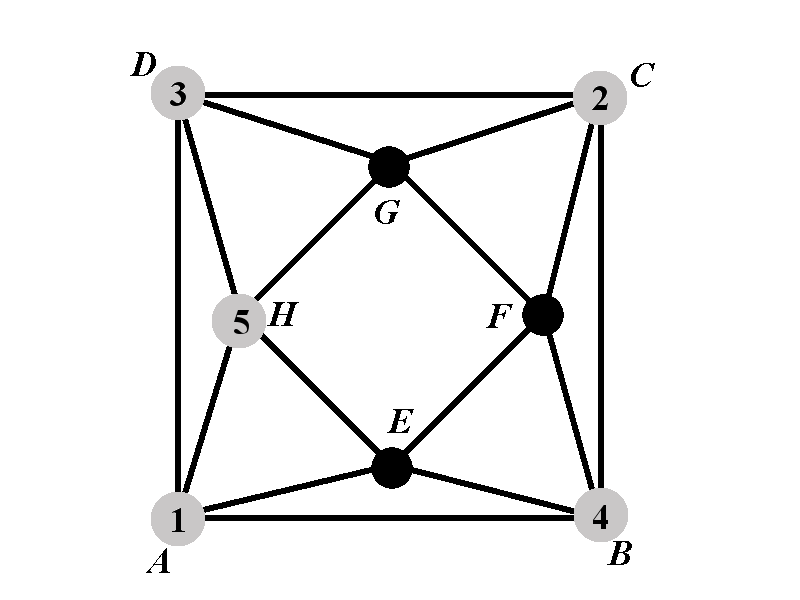Back to: Chromatic Polynomial Case $$2.2^{\circ}$$
##### Case 2.2.2: Calculation of $$P_{2.2.2^{\circ}}(k)$$We will consider cases based on the color of the vertex $$E$$. We know that $$E$$ can‘t have any of the colors $$1$$, $$4$$, and $$5$$. We will consider 3 cases: Case $$2.2.2.1^{\circ}$$ is the one in which $$E$$ has color $$2$$; Case $$2.2.2.2^{\circ}$$ is the one in which $$E$$ has color $$3$$; and Case $$2.2.2.3^{\circ}$$ is the one in which $$E$$ has none of the colors from $$\{1,2,3,4,5\}$$. We will introduce the following notation:

• $$2.2.2.1^{\circ}$$ Denote by $$P_{2.2.2.1^{\circ}}(k)$$ the number of proper colorings of $$\Gamma$$ in which $$A$$, $$B$$, $$C$$, $$D$$, $$E$$, and $$H$$ have the colors $$1$$, $$4$$, $$2$$, $$3$$, $$2$$, and $$5$$, respectively.

• $$2.2.2.2^{\circ}$$ Denote by $$P_{2.2.2.2^{\circ}}(k)$$ the number of proper colorings of $$\Gamma$$ in which $$A$$, $$B$$, $$C$$, $$D$$, $$E$$, and $$H$$ have the colors $$1$$, $$4$$, $$2$$, $$3$$, $$3$$, and $$5$$, respectively.

• $$2.2.2.3^{\circ}$$ Denote by $$P_{2.2.2.3^{\circ}}(k)$$ the number of proper colorings of $$\Gamma$$ in which $$A$$, $$B$$, $$C$$, $$D$$, $$E$$, and $$H$$ have the colors $$1$$, $$4$$, $$2$$, $$3$$, $$6$$, and $$5$$, respectively.

The number $$P_{2.2.2^{\circ}}(k)$$ satisfies $P_{2.2.2^{\circ}}(k)=P_{2.2.2.1^{\circ}}(k)+P_{2.2.2.2^{\circ}}(k)+(k-5)P_{2.2.2.3^{\circ}}(k).$

We can now complete the calculation of $$P_{2.2.2^{\circ}}(k)$$.

\begin{eqnarray*} P_{2.2.2^{\circ}}(k)&=&P_{2.2.2.1^{\circ}}(k)+P_{2.2.2.2^{\circ}}(k)+(k-5)P_{2.2.2.3^{\circ}}(k)\newline &=&k-2+(k-3)(k-4)+k-3+(k-4)^2+(k-5)\left(2(k-3)+(k-4)(k-5)\right)\newline &=&k^3-10k^2+36k-47. \end{eqnarray*}